# sampling

Currently, there are 7 functions associated with the sample verb in the sgsR package:

• sample_srs() - simple random sampling

• sample_systematic()- systematic sampling in a grid or hexagon tessellation

• sample_strat() - stratified sampling within a sraster

• sample_nc() - Nearest centroid sampling. See Melville & Stone (2016)

• sample_clhs() - Latin hypercube sampling

• sample_balanced() - see BalancedSampling

• sample_ahels() - adapted hypercube evaluation of a legacy sample (ahels)

## Access

One key feature of using some sample_* functions is its ability to define access corridors. Users can supply a road access network (must be sf line objects) and define buffers around access where samples should be excluded and included.

Relevant and applicable parameters when access is defined are:

• buff_inner - Can be left as NULL (default). Inner buffer parameter that defines the distance from access where samples cannot be taken (i.e. if you don’t want samples within 50 m of your access layer set buff_inner = 50).

• buff_outer - Outer buffer parameter that defines the maximum distance that the samples can be located from access (i.e. if you don’t want samples more than 200 meters from your access layer set buff_inner = 200).

## sample_srs

We have demonstrated a simple example of using the sample_srs() function in vignette("sgsR"). We will demonstrate additional examples below.

The input required for sample_srs() is a raster. This means that sraster and mraster are supported for this function.

#--- perform simple random sampling ---#
sample_srs(raster = sraster, # input sraster
nSamp = 200, # number of desired samples
plot = TRUE) # plot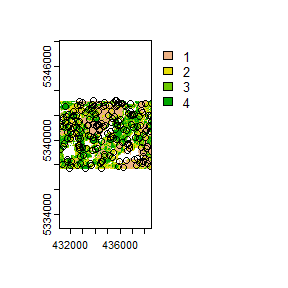#> Simple feature collection with 200 features and 0 fields
#> Geometry type: POINT
#> Dimension:     XY
#> Bounding box:  xmin: 431190 ymin: 5337710 xmax: 438550 ymax: 5343210
#> CRS:           +proj=utm +zone=17 +ellps=GRS80 +units=m +no_defs
#> First 10 features:
#>                  geometry
#> 1  POINT (433850 5341290)
#> 2  POINT (433850 5341290)
#> 3  POINT (434830 5341170)
#> 4  POINT (432250 5341030)
#> 5  POINT (437550 5338210)
#> 6  POINT (432110 5341630)
#> 7  POINT (434290 5340330)
#> 8  POINT (437030 5341970)
#> 9  POINT (438390 5338370)
#> 10 POINT (438330 5341970)
sample_srs(raster = mraster, # input mraster
nSamp = 200, # number of desired samples
access = access, # define access road network
mindist = 200, # minimum distance samples must be apart from one another
buff_inner = 50, # inner buffer - no samples within this distance from road
buff_outer = 200, # outer buffer - no samples further than this distance from road
plot = TRUE) # plot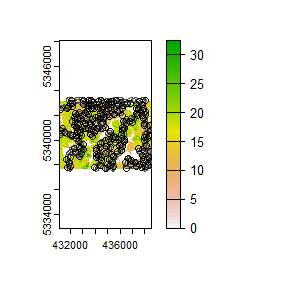#> Simple feature collection with 200 features and 0 fields
#> Geometry type: POINT
#> Dimension:     XY
#> Bounding box:  xmin: 431170 ymin: 5337730 xmax: 438490 ymax: 5343230
#> CRS:           +proj=utm +zone=17 +ellps=GRS80 +units=m +no_defs
#> First 10 features:
#>                  geometry
#> 1  POINT (437570 5343150)
#> 2  POINT (434050 5341850)
#> 3  POINT (438430 5339090)
#> 4  POINT (433230 5341350)
#> 5  POINT (432850 5338950)
#> 6  POINT (432310 5341230)
#> 7  POINT (435830 5339070)
#> 8  POINT (433430 5343210)
#> 9  POINT (435370 5340110)
#> 10 POINT (434410 5341730)

## sample_systematic

The sample_systematic() function applies systematic sampling across an area with the cellsize parameter defining the resolution of the tessellation. The tessellation shape can be modified using the square parameter. Assigning TRUE (default) to the square parameter results in a regular grid and assigning FALSE results in a hexagonal grid. The location of samples can also be adjusted using the locations parameter, where centers takes the center, corners takes all corners, and random takes a random location within each tessellation.

#--- perform grid sampling ---#
sample_systematic(raster = sraster, # input sraster
cellsize = 1000, # grid distance
plot = TRUE) # plot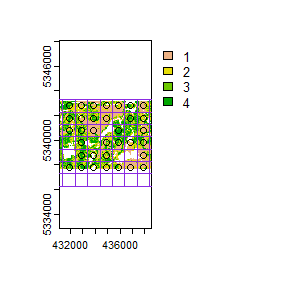#> Simple feature collection with 34 features and 0 fields
#> Geometry type: POINT
#> Dimension:     XY
#> Bounding box:  xmin: 431880 ymin: 5337780 xmax: 437880 ymax: 5342780
#> CRS:           +proj=utm +zone=17 +ellps=GRS80 +units=m +no_defs
#> First 10 features:
#>                  geometry
#> 1  POINT (431880 5337780)
#> 2  POINT (432880 5337780)
#> 3  POINT (433880 5337780)
#> 4  POINT (434880 5337780)
#> 5  POINT (435880 5337780)
#> 6  POINT (436880 5337780)
#> 7  POINT (437880 5337780)
#> 8  POINT (432880 5338780)
#> 9  POINT (433880 5338780)
#> 10 POINT (434880 5338780)
#--- perform grid sampling ---#
sample_systematic(raster = sraster, # input sraster
cellsize = 500, # grid distance
square = FALSE, # hexagonal tessellation
location = "random", # random sample within tessellation
plot = TRUE) # plot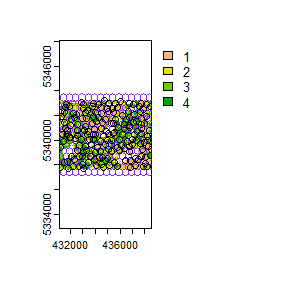#> Simple feature collection with 174 features and 0 fields
#> Geometry type: POINT
#> Dimension:     XY
#> Bounding box:  xmin: 431177.6 ymin: 5337700 xmax: 438546.2 ymax: 5343234
#> CRS:           +proj=utm +zone=17 +ellps=GRS80 +units=m +no_defs
#> First 10 features:
#>                    geometry
#> 1  POINT (431192.3 5337783)
#> 2    POINT (431179 5339447)
#> 3  POINT (431359.8 5340452)
#> 4  POINT (431177.6 5342245)
#> 5  POINT (431492.3 5338177)
#> 6    POINT (431459 5339903)
#> 7  POINT (431609.4 5340923)
#> 8  POINT (431299.9 5341901)
#> 9  POINT (431602.2 5342628)
#> 10 POINT (431794.1 5337700)
sample_systematic(raster = sraster, # input sraster
cellsize = 500, # grid distance
access = access, # define access road network
buff_outer = 200, # outer buffer - no samples further than this distance from road
square = FALSE, # hexagonal tessellation
location = "corners", # take corners instead of centers
plot = TRUE)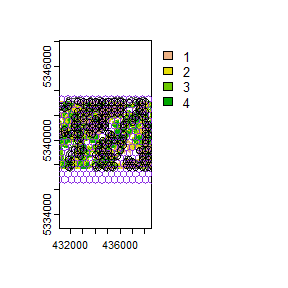#> Simple feature collection with 643 features and 0 fields
#> Geometry type: POINT
#> Dimension:     XY
#> Bounding box:  xmin: 431290 ymin: 5337830 xmax: 438540 ymax: 5343171
#> CRS:           +proj=utm +zone=17 +ellps=GRS80 +units=m +no_defs
#> First 10 features:
#>                  geometry
#> 1  POINT (431290 5340428)
#> 2  POINT (431290 5342738)
#> 3  POINT (431290 5340428)
#> 4  POINT (431540 5340861)
#> 5  POINT (431540 5340573)
#> 6  POINT (431290 5340428)
#> 7  POINT (431290 5342738)
#> 8  POINT (431540 5342594)
#> 9  POINT (431790 5337830)
#> 10 POINT (431790 5338408)

## sample_strat

The sample_strat() contains two methods to perform sampling:

• "Queinnec" - Hierarchical sampling using a focal window to isolate contiguous groups of stratum pixels, which was originally developed by Martin Queinnec.

• "random" - Traditional stratified random sampling. This method ignores much of the functionality of the algorithm to allow users the capability to use standard stratified random sampling approaches without the use of a focal window to locate contiguous stratum cells.

### method = "Queinnec"

Queinnec, M., White, J. C., & Coops, N. C. (2021). Comparing airborne and spaceborne photon-counting LiDAR canopy structural estimates across different boreal forest types. Remote Sensing of Environment, 262(August 2020), 112510.

This algorithm uses moving window (wrow and wcol parameters) to filter the input sraster to prioritize sample locations where stratum pixels are spatially grouped, rather than dispersed individuals across the landscape.

Sampling is performed using 2 rules:

• Rule 1 - Sample within spatially grouped stratum pixels. Moving window defined by wrow and wcol.

• Rule 2 - If no more samples exist to satisfy desired sampling count, individual stratum pixels are sampled.

The rule applied to a select a particular sample is defined in the rule attribute of output samples. We give a few examples below:

#--- perform stratified sampling random sampling ---#
sample_strat(sraster = sraster, # input sraster
nSamp = 200, # desired sample number
plot = TRUE) # plot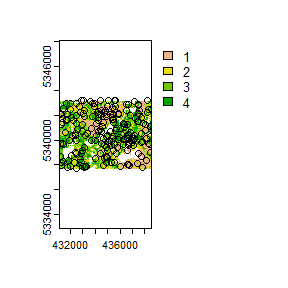#> Simple feature collection with 200 features and 3 fields
#> Geometry type: POINT
#> Dimension:     XY
#> Bounding box:  xmin: 431170 ymin: 5337710 xmax: 438510 ymax: 5343230
#> CRS:           NA
#> First 10 features:
#>    strata type  rule               geometry
#> x       1  new rule1 POINT (434090 5341390)
#> x1      1  new rule2 POINT (436610 5339150)
#> x2      1  new rule2 POINT (436750 5337990)
#> x3      1  new rule2 POINT (438490 5338990)
#> x4      1  new rule2 POINT (437530 5342790)
#> x5      1  new rule2 POINT (435570 5342490)
#> x6      1  new rule2 POINT (433970 5342750)
#> x7      1  new rule2 POINT (434410 5342090)
#> x8      1  new rule2 POINT (433110 5341950)
#> x9      1  new rule2 POINT (433810 5343010)

In some cases, users might want to include existing samples within the algorithm. In order to adjust the total number of samples needed per stratum to reflect those already present in existing, we can use the intermediate function extract_strata().

This function uses the sraster and existing samples and extracts the stratum for each. These samples can be included within sample_strat(), which adjusts total samples required per class based on representation in existing.

#--- extract strata values to existing samples ---#
e.sr <- extract_strata(sraster = sraster, # input sraster
existing = existing) # existing samples to add strata value to

Notice that e.sr now has an attribute named strata. If that parameter is not there, sample_strat() will give an error.

sample_strat(sraster = sraster, # input sraster
nSamp = 200, # desired sample number
access = access, # define access road network
existing = e.sr, # existing samples with strata values
mindist = 200, # minimum distance samples must be apart from one another
buff_inner = 50, # inner buffer - no samples within this distance from road
buff_outer = 200, # outer buffer - no samples further than this distance from road
plot = TRUE) # plot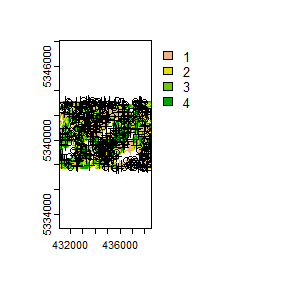#> Simple feature collection with 400 features and 3 fields
#> Geometry type: POINT
#> Dimension:     XY
#> Bounding box:  xmin: 431110 ymin: 5337710 xmax: 438550 ymax: 5343230
#> CRS:           NA
#> First 10 features:
#>    strata     type     rule               geometry
#> 1       1 existing existing POINT (438010 5341070)
#> 2       1 existing existing POINT (435850 5341950)
#> 3       1 existing existing POINT (434070 5340610)
#> 4       1 existing existing POINT (435210 5340550)
#> 5       1 existing existing POINT (436910 5337970)
#> 6       1 existing existing POINT (434330 5341130)
#> 7       1 existing existing POINT (434930 5341670)
#> 8       1 existing existing POINT (437970 5340290)
#> 9       1 existing existing POINT (433470 5340930)
#> 10      1 existing existing POINT (434750 5342750)

As seen on the code in the example above, the defined mindist parameter specifies the minimum euclidean distance that samples must be apart from one another.

Notice that the sample outputs have type and rule attributes which outline whether the samples are existing or new and whether rule1 or rule2 were used to select the individual samples. If type is existing (a user provided existing sample), rule will be existing as well as seen above.

sample_strat(sraster = sraster, # input
nSamp = 200, # desired sample number
access = access, # define access road network
existing = e.sr, # existing samples with strata values
include = TRUE, # include existing plots in nSamp total
buff_outer = 200, # outer buffer - no samples further than this distance from road
plot = TRUE) # plot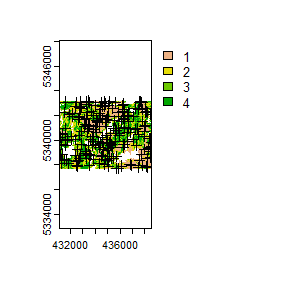#> Simple feature collection with 200 features and 3 fields
#> Geometry type: POINT
#> Dimension:     XY
#> Bounding box:  xmin: 431170 ymin: 5337710 xmax: 438550 ymax: 5343170
#> CRS:           NA
#> First 10 features:
#>    strata     type     rule               geometry
#> 1       1 existing existing POINT (438010 5341070)
#> 2       1 existing existing POINT (435850 5341950)
#> 3       1 existing existing POINT (434070 5340610)
#> 4       1 existing existing POINT (435210 5340550)
#> 5       1 existing existing POINT (436910 5337970)
#> 6       1 existing existing POINT (434330 5341130)
#> 7       1 existing existing POINT (434930 5341670)
#> 8       1 existing existing POINT (437970 5340290)
#> 9       1 existing existing POINT (433470 5340930)
#> 10      1 existing existing POINT (434750 5342750)

The include parameter determines whether existing samples should be included in the total count of samples defined by nSamp. By default, the include parameter is set as FALSE.

### method = "random

Stratified random sampling with equal probability for all cells (using default algorithm values for mindist and no use of access functionality). In essence this method perform the sample_srs algorithm for each stratum separately to meet the specified sample allocation.

#--- perform stratified sampling random sampling ---#
sample_strat(sraster = sraster, # input sraster
method = "random", #stratified random sampling
nSamp = 200, # desired sample number
plot = TRUE) # plot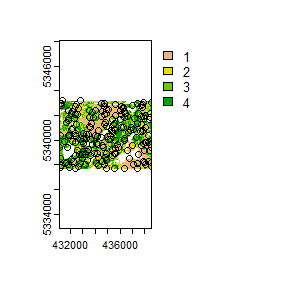#> Simple feature collection with 200 features and 1 field
#> Geometry type: POINT
#> Dimension:     XY
#> Bounding box:  xmin: 431110 ymin: 5337710 xmax: 438530 ymax: 5343230
#> Projected CRS: UTM Zone 17, Northern Hemisphere
#> First 10 features:
#>    strata               geometry
#> 1       1 POINT (434510 5343010)
#> 2       1 POINT (434510 5343010)
#> 3       1 POINT (433530 5341070)
#> 4       1 POINT (434750 5341290)
#> 5       1 POINT (433030 5340530)
#> 6       1 POINT (435850 5339430)
#> 7       1 POINT (437810 5342610)
#> 8       1 POINT (434910 5342150)
#> 9       1 POINT (434110 5339770)
#> 10      1 POINT (434710 5342050)

## sample_nc

sample_nc() function implements the Nearest Centroid sampling algorithm described in Melville & Stone (2016). The algorithm uses kmeans clustering where the number of clusters (centroids) is equal to the desired number of samples (nSamp). Cluster centers are located, which then prompts the nearest neighbour mraster pixel for each cluster to be located (assuming default k parameter). These nearest neighbours are the output samples. Basic usage is as follows:

#--- perform simple random sampling ---#
sample_nc(mraster = mraster, # input
nSamp = 25, # desired sample number
plot = TRUE)
#> K-means being performed on 3 layers with 25 centers.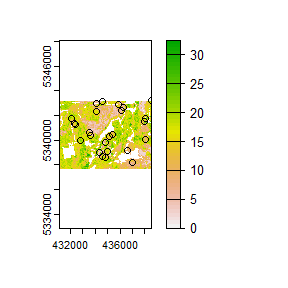#> Simple feature collection with 25 features and 4 fields
#> Geometry type: POINT
#> Dimension:     XY
#> Bounding box:  xmin: 432090 ymin: 5338190 xmax: 438510 ymax: 5343210
#> CRS:           +proj=utm +zone=17 +ellps=GRS80 +units=m +no_defs
#> First 10 features:
#>        zq90 pzabove2  zsd kcenter               geometry
#> 87095 18.00     92.9 3.44       1 POINT (434810 5338570)
#> 63222  6.56     14.0 1.74       2 POINT (434790 5339850)
#> 60884 21.20     91.4 5.53       3 POINT (432790 5339970)
#> 94291  8.38     85.8 1.83       4 POINT (436990 5338190)
#> 59654  4.63     32.6 1.03       5 POINT (438030 5340050)
#> 16935 15.50     94.8 2.71       6 POINT (434090 5342330)
#> 75994  8.69     41.2 2.29       7 POINT (436590 5339170)
#> 54287 14.70     88.8 3.50       8 POINT (435130 5340330)
#> 4999  17.10     88.3 4.24       9 POINT (434090 5342970)
#> 52434 20.80     22.1 6.89      10 POINT (435370 5340430)

Altering the k parameter leads to a multiplicative increase in output samples where total output samples = $$nSamp * k$$.

#--- perform simple random sampling ---#
samples <- sample_nc(mraster = mraster, # input
k = 2, # number of nearest neighbours to take for each kmeans center
nSamp = 25, # desired sample number
plot = TRUE)
#> K-means being performed on 3 layers with 25 centers.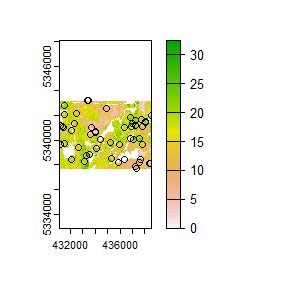#--- total samples = nSamp * k (25 * 2) = 50 ---#
nrow(samples)
#>  50

Visualizing what the kmeans centers and samples nearest neighbours looks like is possible when using details = TRUE. The $kplot output provides a quick visualization of where the centers are based on a scatter plot of the first 2 layers in mraster. Notice that the centers are well distributed in covariate space and chosen samples are the closest pixels to each center (nearest neighbours). #--- perform simple random sampling with details ---# details <- sample_nc(mraster = mraster, # input nSamp = 25, # desired sample number details = TRUE) #> K-means being performed on 3 layers with 25 centers. #--- plot ggplot output ---# details$kplot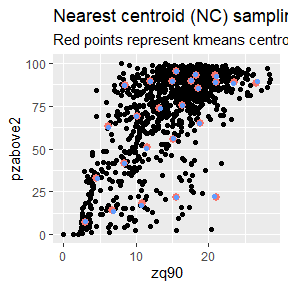## sample_clhs

sample_clhs() function implements conditioned Latin hypercube (clhs) sampling methodology from the clhs package. A number of other functions in the sgsR package help to provide guidance on clhs sampling including calculate_pop() and calculate_lhsOpt(). Check out these functions to better understand how sample numbers could be optimized.

The syntax for this function is similar to others shown above, although parameters like iter, which define the number of iterations within the Metropolis-Hastings process are important to consider. In these examples we use a low iter value because it takes less time to run. Default values for iter within the clhs package are 10,000.

sample_clhs(mraster = mraster, # input
nSamp = 200, # desired sample number
plot = TRUE, # plot
iter = 100) # number of iterations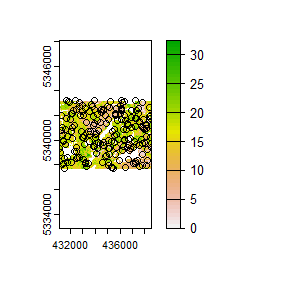sample_clhs(mraster = mraster, # input
nSamp = 300, # desired sample number
iter = 100, # number of iterations
existing = existing, # existing samples
access = access, # define access road network
buff_inner = 100, # inner buffer - no samples within this distance from road
buff_outer = 300, # outer buffer - no samples further than this distance from road
plot = TRUE) # plot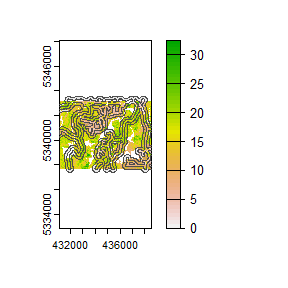The cost parameter defines the mraster covariate, which is used to constrain the clhs sampling. This could be any number of variables. An example could be the distance a pixel is from road access (e.g. from calculate_distance() see example below), terrain slope, the output from calculate_coobs(), or many others.

#--- cost constrained examples ---#
#--- calculate distance to access layer for each pixel in mr ---#
mr.c <- calculate_distance(raster = mraster, # input
access = access,
plot = TRUE) # define access road network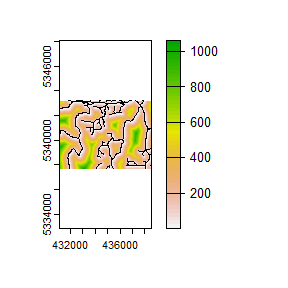sample_clhs(mraster = mr.c, # input
nSamp = 250, # desired sample number
iter = 100, # number of iterations
cost = "dist2access", # cost parameter - name defined in calculate_distance()
plot = TRUE) # plot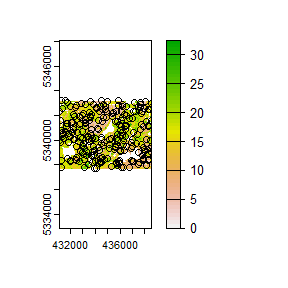## sample_balanced

The sample_balanced() algorithm performs a balanced sampling methodology from the stratifyR / SamplingBigData packages.

sample_balanced(mraster = mraster, # input
nSamp = 200, # desired sample number
plot = TRUE) # plot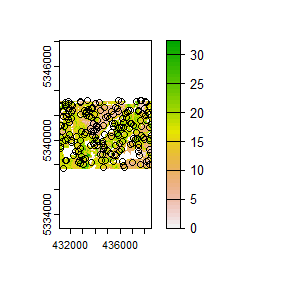#> Simple feature collection with 200 features and 0 fields
#> Geometry type: POINT
#> Dimension:     XY
#> Bounding box:  xmin: 431150 ymin: 5337750 xmax: 438550 ymax: 5343210
#> CRS:           +proj=utm +zone=17 +ellps=GRS80 +units=m +no_defs
#> First 10 features:
#>                  geometry
#> 1  POINT (433330 5343210)
#> 2  POINT (437630 5343210)
#> 3  POINT (437770 5343210)
#> 4  POINT (435890 5343190)
#> 5  POINT (432790 5343170)
#> 6  POINT (437310 5343170)
#> 7  POINT (438030 5343170)
#> 8  POINT (431850 5343150)
#> 9  POINT (436090 5343110)
#> 10 POINT (434310 5343090)
sample_balanced(mraster = mraster, # input
nSamp = 100, # desired sample number
algorithm = "lcube", # algorithm type
access = access, # define access road network
buff_inner = 50, # inner buffer - no samples within this distance from road
buff_outer = 200) # outer buffer - no samples further than this distance from road
#> Simple feature collection with 100 features and 0 fields
#> Geometry type: POINT
#> Dimension:     XY
#> Bounding box:  xmin: 431150 ymin: 5337890 xmax: 438530 ymax: 5343210
#> CRS:           +proj=utm +zone=17 +ellps=GRS80 +units=m +no_defs
#> First 10 features:
#>                  geometry
#> 1  POINT (435290 5341890)
#> 2  POINT (434050 5342610)
#> 3  POINT (431970 5338830)
#> 4  POINT (434430 5338710)
#> 5  POINT (434970 5340570)
#> 6  POINT (431730 5338250)
#> 7  POINT (433150 5342390)
#> 8  POINT (437270 5338150)
#> 9  POINT (433870 5341490)
#> 10 POINT (432650 5339150)

## sample_ahels

The sample_ahels() function performs the adapted Hypercube Evaluation of a Legacy Sample (ahels) algorithm usingexisting sample data and an mraster. New samples are allocated based on quantile ratios between the existing sample and mraster covariate dataset.

This algorithm was adapted from that presented in the paper below, which we highly recommend.

Malone BP, Minansy B, Brungard C. 2019. Some methods to improve the utility of conditioned Latin hypercube sampling. PeerJ 7:e6451 DOI 10.7717/peerj.6451

This algorithm:

1. Determines the quantile distributions of existing samples and mraster covariates.

2. Determines quantiles where there is a disparity between samples and covariates.

3. Prioritizes sampling within those quantile to improve representation.

To use this function, user must first specify the number of quantiles (nQuant) followed by either the nSamp (total number of desired samples to be added) or the threshold (sampling ratio vs. covariate coverage ratio for quantiles - default is 0.9) parameters. We recommended you setting the threshold values at or below 0.9.

sample_ahels(mraster = mraster,
existing = existing, # existing samples
plot = TRUE) # plot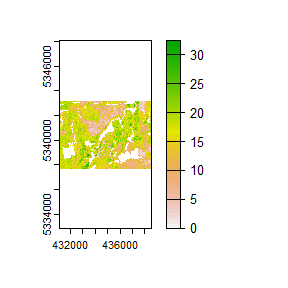#> Simple feature collection with 254 features and 7 fields
#> Geometry type: POINT
#> Dimension:     XY
#> Bounding box:  xmin: 431170 ymin: 5337710 xmax: 438550 ymax: 5343190
#> CRS:           +proj=utm +zone=17 +ellps=GRS80 +units=m +no_defs
#> First 10 features:
#>      type.x  zq90 pzabove2  zsd strata type.y  rule               geometry
#> 1  existing  7.42     80.4 1.57      1    new rule1 POINT (438010 5341070)
#> 2  existing 10.20     92.2 2.39      1    new rule2 POINT (435850 5341950)
#> 3  existing  3.69     11.2 0.87      1    new rule2 POINT (434070 5340610)
#> 4  existing  8.20      3.9 2.36      1    new rule2 POINT (435210 5340550)
#> 5  existing  9.13     82.9 1.93      1    new rule2 POINT (436910 5337970)
#> 6  existing  4.69     19.0 1.22      1    new rule2 POINT (434330 5341130)
#> 7  existing  3.54     46.3 1.21      1    new rule2 POINT (434930 5341670)
#> 8  existing  7.16     62.8 1.70      1    new rule2 POINT (437970 5340290)
#> 9  existing  3.09      6.7 0.58      1    new rule2 POINT (433470 5340930)
#> 10 existing  8.01     30.2 2.07      1    new rule2 POINT (434750 5342750)

Notice that no threshold, nSamp, or nQuant were defined. That is because the default setting for threshold = 0.9 and nQuant = 10.

The first matrix output shows the quantile ratios between the sample and the covariates. A value of 1.0 indicates that samples are represented relative to the quantile coverage. Values > 1.0 indicate over representation of samples, while < 1.0 indicate under representation of samples.

sample_ahels(mraster = mraster,
existing = existing, # existing samples
nQuant = 20, # define 20 quantiles
nSamp = 300) # total samples desired#> Simple feature collection with 500 features and 7 fields
#> Geometry type: POINT
#> Dimension:     XY
#> Bounding box:  xmin: 431110 ymin: 5337710 xmax: 438550 ymax: 5343210
#> CRS:           +proj=utm +zone=17 +ellps=GRS80 +units=m +no_defs
#> First 10 features:
#>      type.x  zq90 pzabove2  zsd strata type.y  rule               geometry
#> 1  existing  7.42     80.4 1.57      1    new rule1 POINT (438010 5341070)
#> 2  existing 10.20     92.2 2.39      1    new rule2 POINT (435850 5341950)
#> 3  existing  3.69     11.2 0.87      1    new rule2 POINT (434070 5340610)
#> 4  existing  8.20      3.9 2.36      1    new rule2 POINT (435210 5340550)
#> 5  existing  9.13     82.9 1.93      1    new rule2 POINT (436910 5337970)
#> 6  existing  4.69     19.0 1.22      1    new rule2 POINT (434330 5341130)
#> 7  existing  3.54     46.3 1.21      1    new rule2 POINT (434930 5341670)
#> 8  existing  7.16     62.8 1.70      1    new rule2 POINT (437970 5340290)
#> 9  existing  3.09      6.7 0.58      1    new rule2 POINT (433470 5340930)
#> 10 existing  8.01     30.2 2.07      1    new rule2 POINT (434750 5342750)

Notice that the total number of samples is 500. This value is the sum of existing samples (200) and number of samples defined by nSamp = 300.